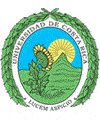``` Universidad de Costa Rica Escuela de Ciencias de la Computación e Informática ```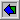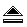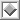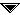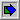# `QuickSort()`

 ```procedure QSORT(m,n) //sort records Rm, ...,Rn into nondecreasing order on key K. Key Km is arbitrarily chosen as the control key. Pointers i and j are used to partition the subfile so that at any time Kl ≤ K, l < i and Kl ≥ K, l > j. It is assumed that Km ≤ Kn+1// if m < n then [i ← m; j ← n + 1; K ← Km loop repeat i ← i + 1 until Ki ≥ K; repeat j ← j - 1 until Kj ≤ K; if i < j then call INTERCHANGE (R(i),R(j)) else exit forever call INTERCHANGE (R(m),R(j)) call QSORT (m, j - 1) call QSORT (j + 1, n)] end QSORT ```
Horowitz, E.; Sahni, S.
"Fundamentals of Data Structures"; Computer Science Press; 1982.

 ``` function partition ( i, j: integer; pivot: keytype ): integer; { partitions A[i], . . . ,A[j] so keys < pivot are at the left and keys ł pivot are on the right. Returns the beginning of the group on the right. } var l, r: integer; { cursors as described above } begin (1) l := i; (2) r := j; repeat (3) swap(A[l], A[r]); { now the scan phase begins } (4) while A[l].key < pivot do (5) l := l + 1; (6) while A[r].key > = pivot do (7) r := r - 1 until (8) l > r; (9) return (l) end; { partition } Fig. 8.13. The procedure partition. ``` ``` procedure quicksort ( i, j: integer ); { sort elements A[i], . . . ,A[j] of external array A } var pivot: keytype; { the pivot value } pivotindex: integer; { the index of an element of A where key is the pivot } k: integer; { beginning index for group of elements ł pivot } begin (1) pivotindex := findpivot(i, j); (2) if pivotindex <> 0 then begin { do nothing if all keys are equal } (3) pivot := A[pivotindex].key; (4) k := partition(i, j, pivot); (5) quicksort(i, k - 1); (6) quicksort(k, j); end end; { quicksort } Fig. 8.14. The procedure quicksort. ```
Aho, Alfred V.; Hopcroft, John E.; Ullman, Jefrrey D.
"Data Structures and Algorithms"; Addisson Wesley Publishing Co.; 1984.